# Zahorski property

(diff) ← Older revision | Latest revision (diff) | Newer revision → (diff)

In his fundamental paper [a4], Z. Zahorski studied (among other topics) zero sets of approximately continuous functions (cf. Approximate continuity; the zero set of a real-valued functionis the set of points at which the value ofis precisely). In modern language, Zahorski proved [a4], Lemma 11, that given a subset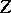of the real line, there is an approximately continuous non-negative bounded functiononsuch that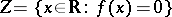if and only if the setis of type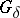(cf. also Set of type()) and closed in the density topology. (Recall that the density topology onis formed by the collection of all Lebesgue measurable sets having each of their points as a density point.)

Notice that the class of approximately continuous functions was introduced by A. Denjoy in [a1] as a generalization of the notion of continuity. It is known that a functionis approximately continuous if and only ifis continuous in the density topology. Functions that are approximately continuous have many pleasing properties. For example, they have the Darboux property and belong to the first Baire class (cf. Baire classes). Moreover, any bounded approximately continuous function is a derivative. Hence Zahorski's theorem can be used in constructing functions with peculiar behaviour. For example, it is easy to construct functions of Pompeiu type: A functiononis a Pompeiu function if it has a bounded derivativeand if the sets on which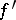is zero or does not vanish, respectively, are both dense in(cf. also Dense set). Also, Zahorski's theorem can serve as a main tool in proving a strengthened form of an old Ward's result from [a3]: Given a set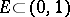of Lebesgue measure zero, there is an approximately continuous functionsuch thatand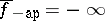on(here,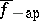, respectively, denote the left-hand upper, respectively right-hand lower, approximative derivative of).

Consider now a metric spaceequipped with another topologywhich is finer than the original metric topology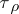. The topologyhas the Luzin–Menshov property with respect toif for each pair of disjoint setswith-closed and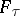-closed, there is a pair of disjoint setswith-open and-open, such that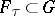and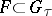. If the topologyhas the Luzin–Menshov property with respect to the metric topology, then it has the Zahorski property: Any-closed subset ofwhich is of the metric typeis the zero set of a bounded-continuous and metric upper semi-continuous function on. Note that, conversely, the Zahorski property does not imply the Luzin–Menshov property. The density topology on the real line has the Luzin–Menshov property. Therefore it has the Zahorski property. Even very general density topologies, or also fine topologies of potential theory, have the Luzin–Menshov property, hence they have the Zahorski property as well.

The Zahorski property can be introduced also in a very general framework of bitopological spaces. By this one understands a setequipped with two topologies. If such a bitopological space satisfies the so-called "binormality condition" , it has the Zahorski property.

A detailed study of the Zahorski property and its applications is given in [a2].

How to Cite This Entry:
Zahorski property. Encyclopedia of Mathematics. URL: http://encyclopediaofmath.org/index.php?title=Zahorski_property&oldid=16247
This article was adapted from an original article by J. LukeÅ¡ (originator), which appeared in Encyclopedia of Mathematics - ISBN 1402006098. See original article